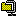### BLOG ARTICLE Rijndael | 2 ARTICLE FOUND

1. 2012.12.10 String encrypters with AES-256 support.
2. 2007.12.19 Interoperable string encrypters for the web. (2)

## String encrypters with AES-256 support.

2012. 12. 10. 01:04RyeolStringEncrypter.zip

This is new string encrypters that is the upgrade of the previous string encrypters to support AES-256. Though PHP and Java are not available, you can download a COM dll (32/64 bit) and a C# class.

The string encrypters take two strings, a key and an initialization vector. To be used as an AES key, the key string is converted into UTF-8, and then is hashed by MD5 or SHA2-256.Similarly, the IV is hashed by MD5.The input string is converted into UTF-8, and then is encrypted using 128-bits or 256-bits AES in Cipher Block Chaining(CBC) with PKCS7 padding. The AES key size depends on the size of the hash for the key string. For example, if the hash is SHA2-256, 256-bits AES is used. Base64 is used for the encrypted binary.The following code is an example of using the string encrypter in C#. The StringEncrypter class is implemented in the StringEncrypter.cs file.

```/*
*  C#
*/
using Ryeol.Security.Cryptography;
...
const string key = "This is a secret key.";
const string iv = "This is an initialization vector.";

// Creates an instance.
StringEncrypter encrypter = new StringEncrypter(key, iv);

// Sets the key hash algorithm. MD5 and SHA2-256 are supported.
// In this case the hash size is 256 bits, AES-256 is used.
encrypter.KeyHashAlgorithm = StringEncrypterKeyHashAlgorithm.SHA2_256;

// Encrypts a string.
string encrypted = encrypter.Encrypt("Test sample");

// Decrypts the encrypted string.
string decrypted = encrypter.Decrypt(encrypted);
```

The following code shows how to use the string encrypter in ASP(VBScript). Since ASP doesn't support built-in AES algorithm, you need to install the RyeolStringEncrypter.dll file which is a COM dll based on XySSL 0.8.
(You also need to install the Visual C++ Redistributable for Visual Studio 2012.)
```'
'  ASP (VBScript)
'
Const conKey = "This is a secret key."
Const conIV = "This is an initialization vector."

' Creates an instance.
Set objEncrypter = Server.CreateObject("Ryeol.StringEncrypter")

objEncrypter.Key = conKey
objEncrypter.IV = conIV

' Sets the key hash algorithm. MD5 and SHA2-256 are supported.
' In this case the hash size is 256 bits, AES-256 is used.
objEncrypter.KeyHashAlgorithm = "SHA2-256"

' Encrypts a string.
strEncrypted = objEncrypter.Encrypt("Test sample")

' Decrypts the encrypted string.
strDecrypted = objEncrypter.Decrypt(strEncrypted)
```

Release Note

June 7, 2013
The C# StringEncrypter has changed to create a hash implementation by using the Create method to support Windows XP.

December 10, 2012
Initial release.
YOUR COMMENT IS THE CRITICAL SUCCESS FACTOR FOR THE QUALITY OF BLOG POST

## Interoperable string encrypters for the web.

2007. 12. 19. 16:01StringEncrypter.zip

A new version is available for ASP and ASP.NET.

I've written string encrypters for ASP, ASP.NET 1.1/2.0, JSP and PHP 5 respectivly, which are interoperable with each other.
The encrypters take two strings, a key and an initial vector. The key and the initial vector are internally converted into UTF-8 and hashed by MD5.String encryption is carried out by using Encrypt method, which first converts the input string into UTF-8 and encodes it according to the PKCS7 padding algorithm (except JSP that uses PKCS5, but it is compatible if the block size is 128bit.) and encrypts it with 128-bit AES algorithm in Cipher Block Chaining(CBC). The encrypted binary is encoded by Base64.
Decrypt method decrypts the encrypted string.The following code is an example of using the string encrypter in ASP.NET 1.1/2.0(C#). The StringEncrypter class is implemented in StringEncrypter.cs.
```/*
*  ASP.NET 1.1/2.0 (C#)
*/
using Hyeongryeol.Security.Cryptography ;
...
const string key = "This is a secret key." ;
const string iv = "This is an initial vector." ;

// Create an instance.
StringEncrypter encrypter = new StringEncrypter (key, iv) ;

// Encrypt a string.
string encrypted = encrypter.Encrypt ("Test sample") ;

// Decrypt a string.
string decrypted = encrypter.Decrypt (encrypted) ;
```
The following code shows how to use the string encrypter in ASP(VBScript). Since ASP doesn't support built-in AES algorithm, you need to install HyeongryeolStringEncrypter.dll which is a COM component based on XySSL 0.8. You have to register it by using the regsvr32.exe program.
(I recommend you to install it in the %WINDOWS%System32 folder.)
```'
'  ASP (VBScript)
'
Const conKey = "This is a secret key."
Const conIV = "This is an initial vector."

' Create an instance.
Set objEncrypter = Server.CreateObject ("Hyeongryeol.StringEncrypter")

objEncrypter.Key = conKey
objEncrypter.InitialVector = conIV

' Encrypt a string.
strEncrypted = objEncrypter.Encrypt ("Test sample")

' Decrypt a string.
strDecrypted = objEncrypter.Decrypt (strEncrypted)
```
The following code is an example in JSP (Java). The StringEncrypter class is implemented in StringEncrypter.java which also requires Base64Encoder.java.
```/*
*  JSP (Java)
*/
import com.hyeongryeol.security.cryptography.*;
...
String key = "This is a secret key.";
String iv = "This is an initial vector.";

// Create an instance.
StringEncrypter encrypter = new StringEncrypter(key, iv);

// Encrypt a string.
String encrypted = encrypter.encrypt("Test sample");

// Decrypt a string.
String decrypted = encrypter.decrypt(encrypted);
```
The following code is an example in PHP 5. The StringEncrypter class is implemented in StringEncrypter.php. It can only be used in a UTF-8 encoded file because UTF-8 encoding and decoding are omitted.
```/*
*  PHP 5 (UTF-8)
*/
require ("StringEncrypter.php") ;

define ("KEY", "This is a secret key.") ;
define ("IV", "This is an initial vector.") ;

// Create an instance.
\$encrypter = new StringEncrypter (KEY, IV) ;

// Encrypt a string.
\$encrypted = \$encrypter->encrypt ("Test sample") ;

// Decrypt a string.
\$decrypted = \$encrypter->decrypt (\$encrypted) ;
```

References
YOUR COMMENT IS THE CRITICAL SUCCESS FACTOR FOR THE QUALITY OF BLOG POST
1. 라이언 2014.12.01 13:13

감사히 잘 사용하고 있습니다.
그런데 이번에 서버를 업그레이드하면서 64비트로 바꿨더니 등록이 안되네요.
64bit 에 맡게 변경하려면 어떻게 해야하나요?
이것저것 해보고 있는데 어렵네요.

부탁드립니다.

2. 64비트안됨 2016.04.05 17:54

안됩니다.